• ## 基尼指数

千次阅读 2018-07-03 11:01:55
基尼指数表示集合D的不确定性，基尼指数Gini(D,A)表示经A=a分割后集合D的不确定性，基尼指数越大，样本的不确定性也就越大，这一点与熵相似。对于给定的样本集合D，其基尼指数为：如果样本集合D根据特征A是否取某一...
分类树可以用基尼指数选择最优特征(CART)，同时决定该特征的最优二值切分点。基尼指数表示集合D的不确定性，基尼指数Gini(D,A)表示经A=a分割后集合D的不确定性，基尼指数越大，样本的不确定性也就越大，这一点与熵相似。对于给定的样本集合D，其基尼指数为：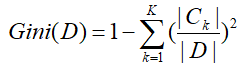如果样本集合D根据特征A是否取某一可能值a被分为两部分，则在特征A的条件下，集合D的基尼指数定义为：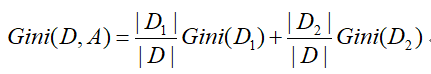展开全文• CART 决策树 [Breiman et al., 1984] 使用"基尼指数" (Gini index)来选择划分属性。CART 是Classification and Regression Tree的简称，这是一种著名的决策树学习算法,分类和回归任务都可用。 一、基尼值和基尼指数...
CART 决策树 [Breiman et al., 1984] 使用"基尼指数" (Gini index)来选择划分属性。CART 是Classification and Regression Tree的简称，这是一种著名的决策树学习算法,分类和回归任务都可用。

一、基尼值和基尼指数

基尼值Gini（D）：从数据集D中随机抽取两个样本，其类别标记不一致的概率。故，Gini（D）值越小，数据集D的纯度越高。

数据集 D 的纯度可用基尼值来度量：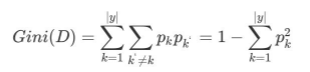其中：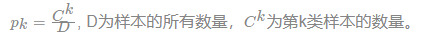基尼指数Gini_index（D）：一般，选择使划分后基尼系数最小的属性作为最优化分属性。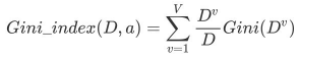二、案例

请根据下图列表，按照基尼指数的划分依据，做出决策树。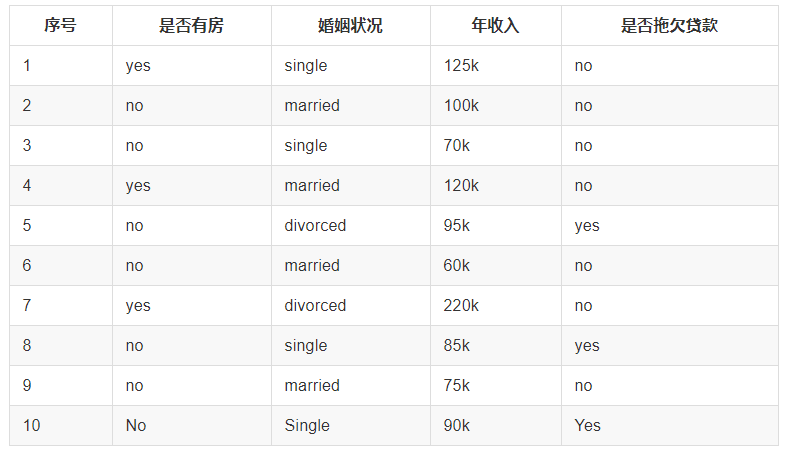1，对数据集非序列标号属性{是否有房，婚姻状况，年收入}分别计算它们的Gini指数，取Gini指数最小的属性作为决策树的根节点属性。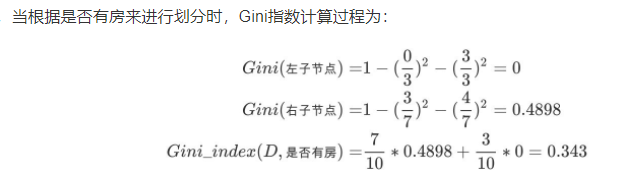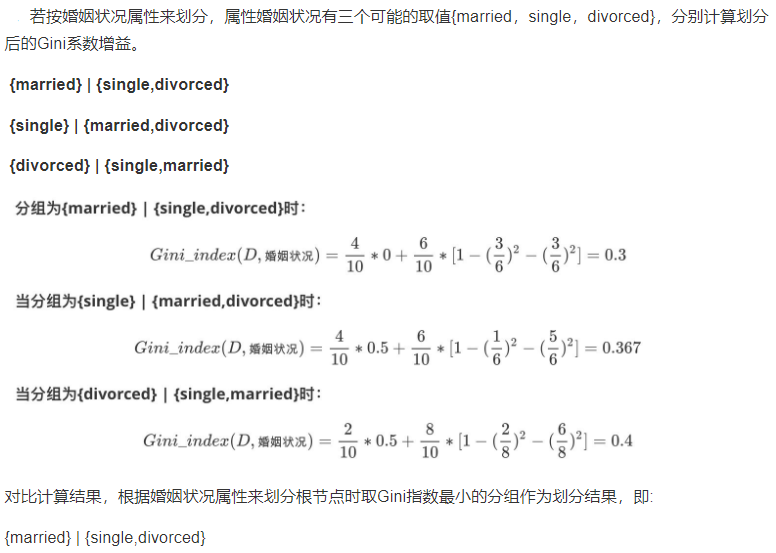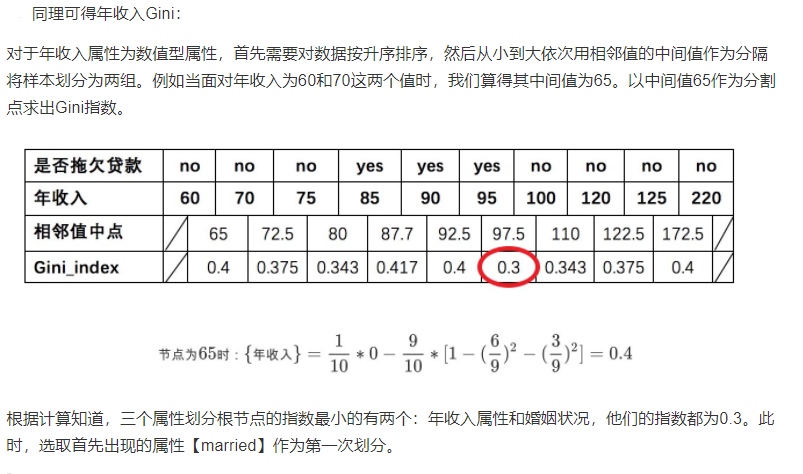2、第二次大循环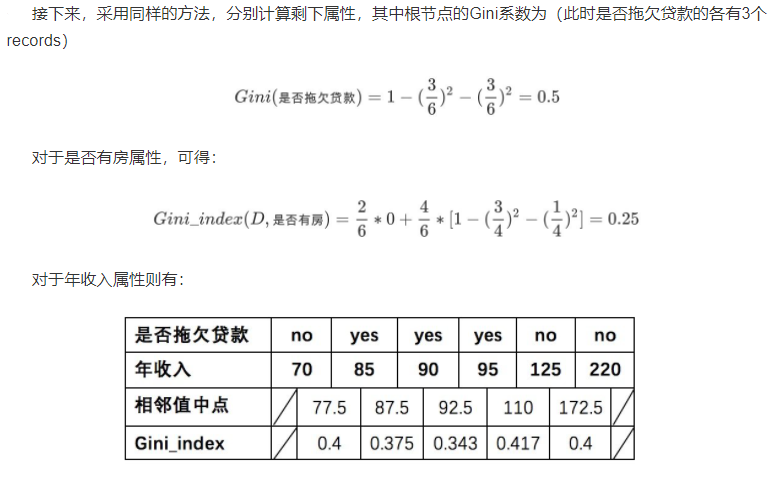3、经过如上流程，构建的决策树，如下图：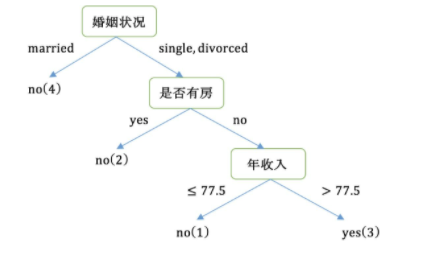现在总结一下CART的算法流程

while(当前节点"不纯")：
1.遍历每个变量的每一种分割方式，找到最好的分割点
2.分割成两个节点N1和N2
end while
每个节点足够“纯”为止


展开全文• 文章目录基尼指数基尼指数简介 基尼指数 基尼指数简介   基尼指数（gini coefficient）代表了模型的不纯度，基尼指数越小，则不纯度越低；基尼指数越大，则不纯度越高，这和信息增益比是相反的。   假设一个训练...
文章目录基尼指数基尼指数简介
基尼指数
基尼指数简介
基尼指数（gini coefficient）代表了模型的不纯度，基尼指数越小，则不纯度越低；基尼指数越大，则不纯度越高，这和信息增益比是相反的。
假设一个训练集有$K$个类别，样本属于第$k$个类别的概率为$p_k$，则它的基尼指数为
$G(p) = \sum_{k=1}^K p_k (1-p_k) = 1 - \sum_{k=1}^K p_k^2$
如果是二分类问题，其中一个分类的概率为$p$，则它的基尼指数为
$G(p) = 2 p(1-p)$
对于某一个训练集$D$，假设训练集有$K$个类别，并且第$k$个类别有$C_k$个样本，则$D$的基尼指数为
$G(D) = 1 - \sum_{k=1}^K ({\frac {|C_k|} {|D|}})^2$
对于某一训练集$D$，如果根据特征$T$的某个特征值是否等于$t$把$D$分成两个子集$D_1$和$D_2$
$D_1 = \{(x,y)\in{D}|A(x)=t\},\quad D_2 = D-D_1$
则$D$的基尼指数为
$G(D,A) = ({\frac {|D_1|} {|D|}})G(D_1) + ({\frac {|D_2|} {|D|}})G(D_2)$
基尼指数$G(D)$表示$D$的不确定性，基尼指数$G(D,A)$表示经过$T=t$分割后$D$的不确定性。基尼指数越大，样本集合的不确定性也就越大，不纯度越高。


展开全文• CART决策树使用“基尼指数”（Gini index）来选择划分属性。书上并没有写出具体的例子供参考，这里给出一个例子。 首先先列出求取基尼指数所需要用的公式。 数据集D的纯度可用基尼值来度量。Gini(D)越小，则数据集D...
CART决策树使用“基尼指数”（Gini index）来选择划分属性。书上并没有写出具体的例子供参考，这里给出一个例子。
首先先列出求取基尼指数所需要用的公式。
数据集D的纯度可用基尼值来度量。Gini(D)越小，则数据集D的纯度越高。（Pk指的是正例在总体中的比例）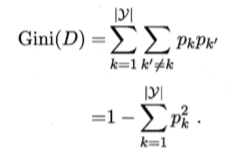属性a的基尼指数定义为：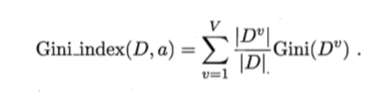求取得出属性a的基尼指数后，再求取其他属性的基尼指数，最后比较获得基尼指数最小的属性为最优划分属性，并继续求取次级最优划分属性，以此类推，直到排序完成。
本例子运用的数据是书上给出的西瓜数据集2.0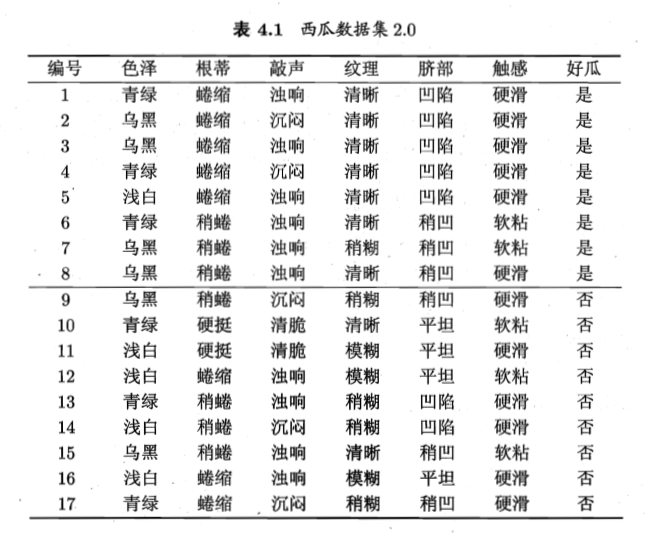这是色泽属性的图示。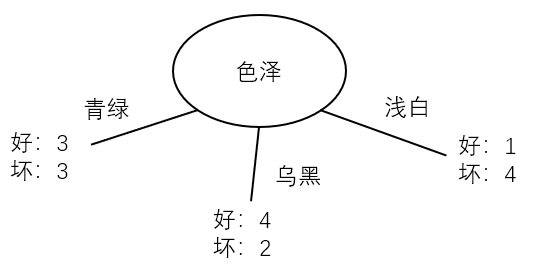根据图示写出程序。
# 求取基尼值
def gini_index_single(a,b):
single_gini = 1 - ((a/(a+b))**2) - ((b/(a+b))**2)
return round(single_gini,4)

# 求取基尼指数
def gini_index(a,b,c,d,e,f):
zuo = gini_index_single(a,b)
zhong = gini_index_single(c,d)
you = gini_index_single(e,f)
sum = a+b+c+d+e+f
gini_index = zuo*((a+b)/sum) + zhong*((c+d)/sum) + you*((e+f)/sum)
return round(gini_index,4)

def gini_index2(a,b,c,d):
zuo = gini_index_single(a,b)
you = gini_index_single(c,d)
sum = a+b+c+d
gini_index2 = zuo*((a+b)/sum) + you*((c+d)/sum)
return round(gini_index2,4)

依次求取色泽、根蒂、敲声、纹理、脐部、触感的基尼指数。如：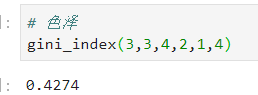通过比较得出，纹理属性的基尼指数最小，为0.3046，为当前的最优划分属性。第一次分叉结束，接下来进行第二次分叉。（此时“纹理”属性不再作为候选划分属性。）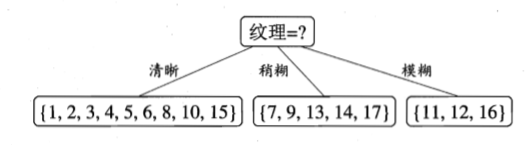以图中第一个分支节点（纹理=“清晰”）为例，计算其他属性的基尼指数。
首先先计算该节点的纯度。（即基尼值）
def gini_index_single(a,b):
single_gini = 1 - ((a/(a+b))**2) - ((b/(a+b))**2)
return round(single_gini,4)
gini_index_single(7,2)

0.3457

此时再计算其他属性的基尼指数，纹理清晰的色泽、根蒂、敲声、脐部、触感的基尼指数分别为0.3333、0.1481、0.1852、0.1481、0.1481。“根蒂”、“脐部”、“触感”三个属性的基尼指数相等，且都比该节点的数值要小（0.3457<0.1481），所以该节点应当继续进行分叉。
此时我们选择使用属性“根蒂”继续进行分叉。当我们使用“蜷缩”属性进行计算时，由于该样本中纹理清晰且根蒂蜷缩的西瓜都是好瓜，所以该节点无法继续进行分叉。（即该节点基尼值为0，没有更小的基尼值了）
def gini_index_single(a,b):
single_gini = 1 - ((a/(a+b))**2) - ((b/(a+b))**2)
return round(single_gini,4)
gini_index_single(5,0)

0.0

我们换用另外一个节点“稍蜷”，发现可以继续进行分叉，继续分叉。
经过计算，最后得到的决策树如下：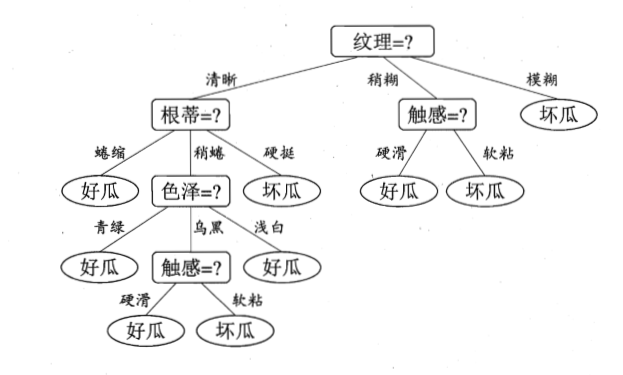展开全文机器学习
• 定义：基尼指数（基尼不纯度）：表示在样本集合中一个随机选中的样本被分错的概率。 注意： Gini指数越小表示集合中被选中的样本被分错的概率越小，也就是说集合的纯度越高，反之，集合越不纯。 即 基尼指数（基尼...机器学习 人工智能 Python
• 在看这篇文章之前如果知道基尼指数的计算公式，就可以接着往下看，如果不知道基尼指数的计算公式，可以看看我之前写的一篇文章决策树的生成–Python代码实现，只需把用Python代码计算基尼指数这部分看完即可 ...
• 1.定义：基尼指数（基尼不纯度）：表示在样本集合中一个随机选中的样本被分错的概率。 注意： Gini指数越小表示集合中被选中的样本被分错的概率越小，也就是说集合的纯度越高，反之，集合越不纯。 def gini_...
• 文中提出了基于基尼指数的分布估计算法，采用实数编码直接对连续随机变量建模，并引入了基尼指数，设计了可以随着进化代数的变化动态调整子代种群的扰动因子函数.实验结果表明,该算法与其他同类算法相比优化精度有了...
• 针对该问题,设计一种基尼指数成本量指导下的抗遮挡光场深度估计方法.首先,利用光场重聚焦方法获得焦栈图像;然后,构造中心视角与其他视角的基尼指数成本量,并根据成本最小原则计算得到初始深度图;最后,结合彩色图进行...
• 经验熵用麦克劳林一阶展开就是基尼指数, 用泰勒展开式在x=1的地方做1阶展开,可以得到相同的结果人工智能
• 基于基尼指数的决策树特征选择算法（CART）及其python实现 基尼指数 与信息增益和增益率类似，基尼指数是另外一种度量指标，由CART决策树使用，其定义如下： 对于二类分类问题,若样本属于正类的概率为 p，则基尼...决策树 机器学习 算法 python
• 根据基尼指数生成决策树时，要注意几个问题： 一、需要判断数据是分类型的还是数值型的，对分类型的拆分子集需判断值是否相等，而对数值型的则需要先计算出区分的值，比较数据与区分值的大小进行拆分。 二、如果是...
• 试将对缺失值的处理机制推广到基尼指数的计算中去 基尼指数的定义 基尼值：用于描述数据集的纯度，Gini(D)越小说明数据集D的纯度越高 其中，p_k是指样本集合D中第k类样本所占的频率。 属性a的基尼指数： 在...
• 基尼指数 定义 是一种不等性度量； 通常用来度量收入不平衡，可以用来度量任何不均匀分布； 是介于0~1之间的数，0-完全相等，1-完全不相等； 总体内包含的类别越杂乱，基尼指数就越大 基尼不纯度指标 在CART...cart算法 机器学习 决策树
• 决策树是一种很基本的分类与回归方法，但正如前面博文机器学习排序算法：RankNet to LambdaRank to LambdaMART中所讲...本文想讨论的是决策树中两个非常重要的决策指标：熵和基尼指数。熵和基尼指数都是用来定义随机...
• 其使用”基尼指数“用来划分属性。 基尼系数（英文：Gini index、Gini Coefficient）是指国际上通用的、用以衡量一个国家或地区居民收入差距的常用指标。 基尼系数最大为“1”，最小等于“0”。基尼系数越接近0表明...决策树 python 机器学习
• 文章目录一，决策树的直观理解二，分类树2.1 信息熵2.2 条件信息熵示例2.3 基尼指数（Gini不纯度）示例 一，决策树的直观理解 二，分类树 2.1 信息熵 信息熵是用来衡量信息不确定性的指标，不确定性是一个事件出现...决策树
• 用信息增益来构建决策树感觉计算量好大啊，下面介绍新的一种构建决策树的方法首先我要引入两个新的概念：基尼系数和基尼指数基尼系数的作用和信息熵的作用相同，都是用来度量数据集的纯度的，公式如下：Pk指的是在...机器学习 决策树
• 基尼系数（Gini index）反映的是从数据集D中随机选取两个样本，其类别标记不一致的概率。因此，基尼系数越小，数据纯度越高。 Gini(D)=1−∑k=1∣γ∣pk2.Gini(D)=1-\sum_{k=1}^{|\gamma|}{p_k^2}.Gini(D)=1−∑k=1...机器学习 决策树
• 决策树（信息熵、信息增益、信息增益率、基尼值和基尼指数、剪枝） 参考自：周志华《机器学习》、”机器学习与数据挖掘“课程 一、概述 决策树(decision tree) 是一类常见的机器学习方法，是基于树结构来进行决策...决策树 机器学习
• Python实现CART(基尼指数) 运行环境 Pyhton3 treePlotter模块(画图所需，不画图可不必) matplotlib(如果使用上面的模块必须) 计算过程 st=>start: 开始 e=>end op1=>operation: 读入数据 op2=>...
• 决策树——信息增益、信息增益率、基尼指数背景——信息量的表示信息熵信息增益增益率基尼系数 这是机器学习课上面教的几个概念，在这里记一下笔记，如果大家发现我的理解有误，请指正，谢谢。 背景——信息量的表示...机器学习 决策树
• 数据及代码地址：https://github.com/qdbszsj/decisionTreeGini这里的代码在信息熵决策树的基础上稍加修改就可以，之前是根据熵增的最大值来确定用哪个属性划分，现在是根据基尼指数（表现数据集D的纯度）的最小值来...
• 文章目录信息增益（ID3算法）信息增益比率（C4.5算法）基尼指数（CART算法） 数据： 信息增益（ID3算法） 信息熵表示信息的混乱程度，熵越大数据越混乱。分类的目的是为了使同一类别的数据尽可能“纯净”，因此追求...决策树 机器学习
•  本文主要介绍了熵，信息增益，信息增益率和基尼指数的基本概念，并使用数据样例进行了计算。 数据样例 名称 是否用鳃呼吸 有无鱼鳍 是否为鱼 鲨鱼 是 有 是 鲫鱼 是 有 是 河蚌 是 无 ...
• 由此我提出了一个疑问：基尼指数和信息增益是等价的吗？ 如果等价，那干嘛还要两个算法？如果不等价，为什么生成的树总是一样的呢？ 二者比较 直接取iris数据集中的一部分作为训练集，并指定一个属性...决策树 信息增益 数据挖掘
• 熵(Entropy) 是度量样本集合纯度最常用的一种指标。 ID3决策树算法就是利用信息增益作为划分数据集的一种方法。 ID3的后继C4.5使用一种称为增益率（Gain Ratio) 的信息增益扩充。...CART中采用基尼指数来进行特征选择。数据挖掘 决策树
• 给定节点t： 这里的Pi(t) 是 类i的...计算单个节点基尼系数： 对于第一组： P(C1) = 0/6 = 0 P(C2) = 6/6 = 1 Gini = 1 – P(C1)2 – P(C2)2 = 1 – 0 – 1 = 0 对于第二组： P(C1) = 1/6 P(C2) = 5/6 Gini = 1 –机器学习...